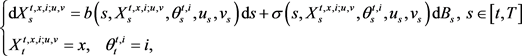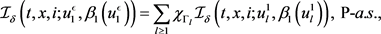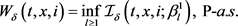# 具有状态切换的二人零和随机微分对策的动态规划原理Dynamic Programming Principles for Two-Player Zero-Sum Stochastic Differential Games with Regime Switching

DOI: 10.12677/PM.2021.114079, PDF, HTML, XML, 下载: 28  浏览: 51

Abstract: In this paper, we study dynamic programming principles for two-player zero-sum stochastic dif-ferential games with regime switching. The main results of this paper concern dynamic program-ming principles with the help of the theory of backward stochastic differential equations.

1. 引言

2. 准备工作

$\left(\Omega ,\mathcal{F},ℙ\right)$ 是一个固定概率空间，其上定义了d维布朗运动 ${\left\{{B}_{s}\right\}}_{s\in \left[0,T\right]}$ 和马氏链 ${\left\{{\theta }_{s}\right\}}_{s\in \left[0,T\right]}$，其中 $\Omega$ 是从 $\left[0,T\right]$${\text{R}}^{d}$ 的连续函数的集合，从0开始 $\left(\Omega ={C}_{0}\left(\left[0,T\right],{\text{R}}^{d}\right)\right)$$\mathcal{F}$$\Omega$ 上完备的Borel $\sigma$ -代数，P是维纳测度。这里我们假设 $\mathcal{F}={\left({\mathcal{F}}_{s}\right)}_{s\in \left[0,T\right]}$，其中 ${\mathcal{F}}_{s}\triangleq {\mathcal{F}}_{s}^{\theta }\vee {\mathcal{F}}_{s}^{B}$。令 ${\left({\mathcal{F}}_{s}^{B}\right)}_{s\in \left[0,T\right]}$ 表示布朗运动 ${\left\{{B}_{s}\right\}}_{s\in \left[0,T\right]}$ 产生的过滤。且 ${\left({\mathcal{F}}_{s}^{\theta }\right)}_{s\in \left[0,T\right]}$ 表示马氏链 ${\left\{{\theta }_{s}\right\}}_{s\in \left[0,T\right]}$ 生成的过滤。假设B和 ${\theta }_{\cdot }$ 是独立的。马氏链 ${\left\{{\theta }_{s}\right\}}_{s\in \left[0,T\right]}$ 取有限状态空间 $\mathcal{M}=\left\{1,\cdots ,m\right\}$ 中的值，是可观测的。马氏链 ${\left\{{\theta }_{s}\right\}}_{s\in \left[0,T\right]}$ 的生成元 $Q={\left({q}_{ik}\right)}_{i,k\in \mathcal{M}}\in {\text{R}}^{m×m}$ 由下式给出

$\text{P}\left({\theta }_{s+\delta }=k|{\theta }_{s}=i,s\in \left[0,T\right]\right)=\left\{\begin{array}{ll}{q}_{ik}\delta +\text{o}\left(\delta \right),\hfill & k\ne i,\hfill \\ 1+{q}_{ii}\delta +\text{o}\left(\delta \right),\hfill & k=i,\hfill \end{array}$(2.1)

(H2.1)

(i) 对于每一固定 $x\in {\text{R}}^{n},i\in \mathcal{M}$$b\left(\cdot ,x,i,\cdot ,\cdot \right)$$\sigma \left(\cdot ,x,i,\cdot ,\cdot \right)$ 是关于 $\left(t,u,v\right)$ 连续的；

(ii) 对于任意 $x,{x}^{\prime }\in {\text{R}}^{n},i\in \mathcal{M}$$u\in U,v\in V$，存在一正的常数C使得，

$|b\left(t,x,i,u,v\right)-b\left(t,{x}^{\prime },i,u,v\right)|+|\sigma \left(t,x,i,u,v\right)-\sigma \left(t,{x}^{\prime },i,u,v\right)|\le C|x-{x}^{\prime }|.$

$|b\left(t,x,i,u,v\right)|+|\sigma \left(t,x,i,u,v\right)|\le C\left(1+|x|\right).$

$\begin{array}{l}E\left\{\underset{t\le s\le T}{\mathrm{sup}}{|{X}_{s}^{t,x,i;u,v}|}^{2}|{\mathcal{F}}_{t}\right\}\le C\left(1+{|x|}^{2}\right),\text{\hspace{0.17em}}\text{P-}a.s.,\\ E\left\{\underset{t\le s\le T}{\mathrm{sup}}{|{X}_{s}^{t,x,i;u,v}-{X}_{s}^{t,{x}^{\prime },i;u,v}|}^{2}|{\mathcal{F}}_{t}\right\}\le C{|x-{x}^{\prime }|}^{2},\text{\hspace{0.17em}}\text{P-}a.s.\end{array}$

${Y}_{s}^{t,x,i;u,v}=\Phi \left({X}_{T}^{t,x,i;u,v},{\theta }_{T}^{t,i}\right)+{\int }_{s}^{T}f\left(r,{X}_{r}^{t,x,i;u,v},{Y}_{r}^{t,x,i;u,v},{Z}_{r}^{t,x,i;u,v},{\theta }_{r}^{t,i},{u}_{r},{v}_{r}\right)\text{d}r-{\int }_{s}^{T}{Z}_{r}^{t,x,i,u,v}\text{d}{B}_{r},\text{ }s\in \left[t,T\right],$ (2.2)

(H2.2)

(i) 对于任意固定的 $\left(x,y,z,i\right)\in {\text{R}}^{n}×\text{R}×{\text{R}}^{d}×\mathcal{M}$$f\left(\cdot ,x,y,z,i,\cdot ,\cdot \right)$ 是关于 $\left(t,u,v\right)$ 连续的。

(ii) 存在 $C>0$ 使得，对于所有 $t\in \left[0,T\right]$$x,{x}^{\prime }\in {\text{R}}^{n}$$y,{y}^{\prime }\in \text{R}$$z,{z}^{\prime }\in {\text{R}}^{d}$$i\in \mathcal{M}$$u\in U$$v\in V$

$|f\left(t,x,y,z,i,u,v\right)-f\left(t,{x}^{\prime },{y}^{\prime },{z}^{\prime },i,u,v\right)|\le C\left(|x-{x}^{\prime }|+|y-{y}^{\prime }|+|z-{z}^{\prime }|\right).$

(iii) 存在 $C>0$ 使得，对于所有的 $x,{x}^{\prime }\in {\text{R}}^{n}$$i\in \mathcal{M}$

$|\Phi \left(x,i\right)-\Phi \left({x}^{\prime },i\right)|\le C|x-{x}^{\prime }|.$(2.3)

$J\left(t,x,i;u,v\right)=E\left[{Y}_{t}^{t,x,i;u,v}|{\mathcal{F}}_{t}\right],\text{\hspace{0.17em}}\left(t,x,i\right)\in \left[0,T\right]×{\text{R}}^{n}×\mathcal{M},$

$W\left(t,x,i\right)=\underset{\beta \in {\mathcal{B}}_{t,T}}{\text{essinf}}\underset{u\in {U}_{t,T}}{\text{esssup}}J\left(t,x,i;u,\beta \left(u\right)\right),$

$U\left(t,x,i\right)=\underset{\alpha \in {\mathcal{A}}_{t,T}}{\text{esssup}}\underset{v\in {V}_{t,T}}{\text{essinf}}J\left(t,x,i;\alpha \left(v\right),v\right).$

$|W\left(t,x,i\right)|\le C\left(1+|x|\right).$

$|W\left(t,x,i\right)-W\left({t}^{\prime },x,i\right)|\le C\left(1+|x|\right){|t-{t}^{\prime }|}^{\frac{1}{2}},$

$|W\left(t,x,i\right)-W\left(t,{x}^{\prime },i\right)|\le C|x-{x}^{\prime }|,$

3. 动态规划原理

${G}_{t,t+\delta }^{t,x,i;u,v}\left[\eta \right]={\stackrel{¯}{{Y}_{t}}}^{t,x,i;u,v}$

${\stackrel{¯}{Y}}_{s}^{t,x,i;u,v}=\eta +{\int }_{s}^{t+\delta }f\left(r,{X}_{r}^{t,\xi ,i;u,v},{\stackrel{¯}{Y}}_{r}^{t,\xi ,i;u,v},{\stackrel{¯}{Z}}_{r}^{t,\xi ,i;u,v},{\theta }_{r}^{t,i},{u}_{r},{v}_{r}\right)\text{d}r-{\int }_{s}^{t+\delta }{\stackrel{¯}{Z}}_{r}^{t,\xi ,i;u,v}\text{d}{B}_{r},\text{ }\text{P-}a.s.,\text{ }s\in \left[t,t+\delta \right],$

${X}^{t,x,i;u,v}$ 是随机微分方程(2.1)的解。根据倒向随机微分方程解的唯一性，我们得到倒向随机微分方程(2.2)的解 ${Y}^{t,x,i;u,v}$ 满足

$\begin{array}{c}J\left(t,x,i;u,v\right)=E\left[{Y}_{t}^{t,x,i;u,v}|{\mathcal{F}}_{t}\right]=E\left[{G}_{t,T}^{t,x,i;u,v}\left[\Phi \left({X}_{T}^{t,x,i;u,v},{\theta }_{T}^{t,i}\right)\right]|{\mathcal{F}}_{t}\right]\\ =E\left[{G}_{t,t+\delta }^{t,x,i;u,v}\left[{Y}_{t+\delta }^{t,x,i;u,v}\right]|{\mathcal{F}}_{t}\right]=E\left[{G}_{t,t+\delta }^{t,x,i;u,v}\left[E\left[{Y}_{t+\delta }^{t,x,i;u,v}|{\mathcal{F}}_{t+\delta }\right]\right]|{\mathcal{F}}_{t}\right]\\ =E\left[{G}_{t,t+\delta }^{t,x,i;u,v}\left[J\left(t+\delta ,{X}_{t+\delta }^{t,x,i;u,v},{\theta }_{t+\delta }^{t,i};u,v\right)\right]|{\mathcal{F}}_{t}\right].\end{array}$

$U\left(t,x,i\right)=\underset{\alpha \in {\mathcal{A}}_{t,t+\delta }}{\text{esssup}}\underset{v\in {V}_{t,t+\delta }}{\text{essinf}}E\left[{G}_{t,t+\delta }^{t,x,i;\alpha \left(v\right),v}\left[U\left(t+\delta ,{X}_{t+\delta }^{t,x,i;\alpha \left(v\right),v},{\theta }_{t+\delta }^{t,i}\right)\right]|{\mathcal{F}}_{t}\right],$

$W\left(t,x,i\right)=\underset{\beta \in {\mathcal{B}}_{t,t+\delta }}{\text{essinf}}\underset{u\in {U}_{t,t+\delta }}{\text{esssup}}E\left[{G}_{t,t+\delta }^{t,x,i;u,\beta \left(u\right)}\left[W\left(t+\delta ,{X}_{t+\delta }^{t,x,i;u,\beta \left(u\right)},{\theta }_{t+\delta }^{t,i}\right)\right]|{\mathcal{F}}_{t}\right].$

${W}_{\delta }\left(t,x,i\right)=\underset{\beta \in {\mathcal{B}}_{t,t+\delta }}{\text{essinf}}\underset{u\in {U}_{t,t+\delta }}{\text{esssup}}E\left[{G}_{t,t+\delta }^{t,x,i;u,\beta \left(u\right)}\left[W\left(t+\delta ,{X}_{t+\delta }^{t,x,i;u,\beta \left(u\right)},{\theta }_{t+\delta }^{t,i}\right)\right]|{\mathcal{F}}_{t}\right].$

${\beta }_{1}\left({u}_{1}\right)=\beta \left({u}_{1}\oplus {u}_{2}\right){|}_{\left[t,t+\delta \right]},\text{\hspace{0.17em}}\text{\hspace{0.17em}}{u}_{1}\in {U}_{t,t+\delta },$

${W}_{\delta }\left(t,x,i\right)\le \underset{{u}_{1}\in {U}_{t,t+\delta }}{\text{esssup}}E\left[{G}_{t,t+\delta }^{t,x,i;{u}_{1},{\beta }_{1}\left({u}_{1}\right)}\left[W\left(t+\delta ,{X}_{t+\delta }^{t,x,i;{u}_{1},{\beta }_{1}\left({u}_{1}\right)},{\theta }_{t+\delta }^{t,i}\right)\right]|{\mathcal{F}}_{t}\right],$

${\mathcal{I}}_{\delta }\left(t,x,i;u,v\right)=E\left[{G}_{t,t+\delta }^{t,x,i;u,v}\left[W\left(t+\delta ,{X}_{t+\delta }^{t,x,i;u,v},{\theta }_{t+\delta }^{t,i}\right)\right]|{\mathcal{F}}_{t}\right]$ 且对于一些序列 $\left\{{u}_{l}^{1},l\ge 1\right\}\subset {U}_{t,t+\delta }$ 使得，

${\mathcal{I}}_{\delta }\left(t,x,i;{\beta }_{1}\right)=\underset{{u}_{1}\in {U}_{t,t+\delta }}{\text{esssup}}{\mathcal{I}}_{\delta }\left(t,x,i;{u}_{1},{\beta }_{1}\left({u}_{1}\right)\right)=\underset{l\ge 1}{\mathrm{sup}}{\mathcal{I}}_{\delta }\left(t,x,i;{u}_{l}^{1},{\beta }_{1}\left({u}_{l}^{1}\right)\right),\text{ }\text{P-}a.s.$

$ϵ>0$ 且设 ${\stackrel{˜}{\Gamma }}_{l}=\left\{{\mathcal{I}}_{\delta }\left(t,x,i;{\beta }_{1}\right)\le {\mathcal{I}}_{\delta }\left(t,x,i;{u}_{l}^{1},{\beta }_{1}\left({u}_{l}^{1}\right)\right)+ϵ\right\}\in {\mathcal{F}}_{t},l\ge 1$。构造 ${\Gamma }_{1}={\stackrel{˜}{\Gamma }}_{1}$${\Gamma }_{l}={\stackrel{˜}{\Gamma }}_{l}\setminus \left(\underset{k=1}{\overset{l-1}{\cup }}{\stackrel{˜}{\Gamma }}_{k}\right)\in {\mathcal{F}}_{t},l\ge 2$${\left\{{\Gamma }_{l}\right\}}_{l\ge 1}$$\left(\Omega ,{\mathcal{F}}_{t}\right)$ 的一分割，且 ${u}_{1}^{ϵ}=\underset{l\ge 1}{\sum }\text{ }\text{ }{\chi }_{{\Gamma }_{l}}{u}_{l}^{1}\in {U}_{t,t+\delta }$。此外，从 ${\beta }_{1}$ 的非预测性，我们得到 ${\beta }_{1}\left({u}_{1}^{ϵ}\right)=\underset{l\ge 1}{\sum }{\chi }_{{\Gamma }_{l}}{\beta }_{1}\left({u}_{l}^{1}\right)$。由倒向随机微分方程的存在性和唯一性，得到对于 ${\beta }_{1}\in {\mathcal{B}}_{t,t+\delta }$$\begin{array}{c}{W}_{\delta }\left(t,x,i\right)\le {\mathcal{I}}_{\delta }\left(t,x,i;{\beta }_{1}\right)\\ \le \underset{l\ge 1}{\sum }{\chi }_{{\Gamma }_{l}}{\mathcal{I}}_{\delta }\left(t,x,i;{u}_{l}^{1},{\beta }_{1}\left({u}_{l}^{1}\right)\right)+ϵ\\ ={\mathcal{I}}_{\delta }\left(t,x,i;{u}_{1}^{ϵ},{\beta }_{1}\left({u}_{1}^{ϵ}\right)\right)+ϵ\\ =E\left[{G}_{t,t+\delta }^{t,x,i;{u}_{1}^{ϵ},{\beta }_{1}\left({u}_{1}^{ϵ}\right)}\left[W\left(t+\delta ,{X}_{t+\delta }^{t,x,i;{u}_{1}^{ϵ},{\beta }_{1}\left({u}_{1}^{ϵ}\right)},{\theta }_{t+\delta }^{t,i}\right)\right]|{\mathcal{F}}_{t}\right]+ϵ,\text{\hspace{0.17em}}\text{P-}a.s.\end{array}$ (3.1)

$W\left(t+\delta ,y,j\right)\le \underset{{u}_{2}\in {U}_{t+\delta ,T}}{\text{esssup}}J\left(t+\delta ,y,j;{u}_{2},{\beta }_{2}\left({u}_{2}\right)\right),\text{\hspace{0.17em}}\text{P-}a.s.$

(i) $|W\left(t+\delta ,y,j\right)-W\left(t+\delta ,{y}^{\prime },j\right)|\le C|y-{y}^{\prime }|,$

(ii) $|J\left(t+\delta ,y,j;{u}_{2},{\beta }_{2}\left({u}_{2}\right)\right)-J\left(t+\delta ,{y}^{\prime },j;{u}_{2},{\beta }_{2}\left({u}_{2}\right)\right)|\le C|y-{y}^{\prime }|,\text{\hspace{0.17em}}\text{P-}a.s.$ (3.2)

$W\left(t+\delta ,{X}_{t+\delta }^{t,x,i;{u}_{1}^{ϵ},{\beta }_{1}\left({u}_{1}^{ϵ}\right)},{\theta }_{t+\delta }^{t,i}\right)\le \underset{{u}_{2}\in {U}_{t+\delta ,T}}{\text{esssup}}J\left(t+\delta ,{X}_{t+\delta }^{t,x,i;{u}_{1}^{ϵ},{\beta }_{1}\left({u}_{1}^{ϵ}\right)},{\theta }_{t+\delta }^{t,i};{u}_{2},{\beta }_{2}\left({u}_{2}\right)\right),\text{\hspace{0.17em}}\text{P-}a.s.$

$\underset{{u}_{2}\in {U}_{t+\delta ,T}}{\text{esssup}}J\left(t+\delta ,{X}_{t+\delta }^{t,x,i;{u}_{1}^{ϵ},{\beta }_{1}\left({u}_{1}^{ϵ}\right)},{\theta }_{t+\delta }^{t,i};{u}_{2},{\beta }_{2}\left({u}_{2}\right)\right)=\underset{k\ge 1}{\mathrm{sup}}J\left(t+\delta ,{X}_{t+\delta }^{t,x,i;{u}_{1}^{ϵ},{\beta }_{1}\left({u}_{1}^{ϵ}\right)},{\theta }_{t+\delta }^{t,i},{u}_{k}^{2},{\beta }_{2}\left({u}_{k}^{2}\right)\right),\text{P-}a.s.$

$ϵ>0$

$\begin{array}{l}{\stackrel{˜}{△}}_{k}=\left\{\underset{{u}_{2}\in {U}_{t+\delta ,T}}{\text{esssup}}J\left(t+\delta ,{X}_{t+\delta }^{t,x,i;{u}_{1}^{ϵ},{\beta }_{1}\left({u}_{1}^{ϵ}\right)},{\theta }_{t+\delta }^{t,i};{u}_{2},{\beta }_{2}\left({u}_{2}\right)\right)\\ \text{\hspace{0.17em}}\text{\hspace{0.17em}}\text{\hspace{0.17em}}\text{\hspace{0.17em}}\text{\hspace{0.17em}}\text{\hspace{0.17em}}\text{ }\le J\left(t+\delta ,{X}_{t+\delta }^{t,x,i;{u}_{1}^{ϵ},{\beta }_{1}\left({u}_{1}^{ϵ}\right)},{\theta }_{t+\delta }^{t,i};{u}_{k}^{2},{\beta }_{2}\left({u}_{k}^{2}\right)\right)+ϵ\right\}\in {\mathcal{F}}_{t+\delta },k\ge 1.\end{array}$

$\begin{array}{l}J\left(t+\delta ,{X}_{t+\delta }^{t,x,i;{u}_{1}^{ϵ},{\beta }_{1}\left({u}_{1}^{ϵ}\right)},{\theta }_{t+\delta }^{t,i};{u}_{2}^{ϵ},{\beta }_{2}\left({u}_{2}^{ϵ}\right)\right)\\ =E\left[{Y}_{t+\delta }^{t+\delta ,{X}_{t+\delta }^{t,x,i;{u}_{1}^{ϵ},{\beta }_{1}\left({u}_{1}^{ϵ}\right)},{\theta }_{t+\delta }^{t,i};{u}_{2}^{ϵ},{\beta }_{2}\left({u}_{2}^{ϵ}\right)}|{\mathcal{F}}_{t+\delta }\right]\\ =E\left[\underset{k\ge 1}{\sum }{\chi }_{{△}_{k}}{Y}_{t+\delta }^{t+\delta ,{X}_{t+\delta }^{t,x,i;{u}_{1}^{ϵ},{\beta }_{1}\left({u}_{1}^{ϵ}\right)},{\theta }_{t+\delta }^{t,i};{u}_{k}^{ϵ},{\beta }_{2}\left({u}_{k}^{ϵ}\right)}|{\mathcal{F}}_{t+\delta }\right]\\ =\underset{k\ge 1}{\sum }{\chi }_{{△}_{k}}J\left(t+\delta ,{X}_{t+\delta }^{t,x,i;{u}_{1}^{ϵ},{\beta }_{1}\left({u}_{1}^{ϵ}\right)},{\theta }_{t+\delta }^{t,i};{u}_{k}^{ϵ},{\beta }_{2}\left({u}_{k}^{ϵ}\right)\right),\text{P-}a.s.\end{array}$

$\begin{array}{l}W\left(t+\delta ,{X}_{t+\delta }^{t,x,i;{u}_{1}^{ϵ},{\beta }_{1}\left({u}_{1}^{ϵ}\right)},{\theta }_{t+\delta }^{t,i}\right)\\ \le \underset{{u}_{2}\in {U}_{t+\delta ,T}}{\text{esssup}}J\left(t+\delta ,{X}_{t+\delta }^{t,x,i;{u}_{1}^{ϵ},{\beta }_{1}\left({u}_{1}^{ϵ}\right)},{\theta }_{t+\delta }^{t,i};{u}_{2},{\beta }_{2}\left({u}_{2}\right)\right)\\ \le E\left[\underset{k\ge 1}{\sum }{\chi }_{{△}_{k}}{Y}_{t+\delta }^{t,x,i;{u}_{1}^{ϵ}\oplus {u}_{k}^{2},\beta \left({u}_{1}^{ϵ}\oplus {u}_{k}^{2}\right)}|{\mathcal{F}}_{t+\delta }\right]+ϵ\\ =E\left[{Y}_{t+\delta }^{t,x,i;{u}_{1}^{ϵ}\oplus {u}_{2}^{ϵ},\beta \left({u}_{1}^{ϵ}\oplus {u}_{2}^{ϵ}\right)}|{\mathcal{F}}_{t+\delta }\right]+ϵ\\ =E\left[{Y}_{t+\delta }^{t,x,i;{u}^{ϵ},\beta \left({u}^{ϵ}\right)}|{\mathcal{F}}_{t+\delta }\right]+ϵ,\text{P-}a.s.,\end{array}$ (3.3)

$\begin{array}{c}{W}_{\delta }\left(t,x,i\right)\le E\left[{G}_{t,t+\delta }^{t,x,i;{u}_{1}^{ϵ},{\beta }_{1}\left({u}_{1}^{ϵ}\right)}\left[E\left[{Y}_{t+\delta }^{t,x,i;{u}^{ϵ},\beta \left({u}^{ϵ}\right)}|{\mathcal{F}}_{t+\delta }\right]+ϵ\right]|{\mathcal{F}}_{t}\right]\\ \le E\left[{G}_{t,t+\delta }^{t,x,i;{u}_{1}^{ϵ},{\beta }_{1}\left({u}_{1}^{ϵ}\right)}\left[E\left[{Y}_{t+\delta }^{t,x,i;{u}^{ϵ},\beta \left({u}^{ϵ}\right)}|{\mathcal{F}}_{t+\delta }\right]\right]|{\mathcal{F}}_{t}\right]+\left(C+1\right)ϵ\\ =E\left[{G}_{t,t+\delta }^{t,x,i;{u}^{ϵ},\beta \left({u}^{ϵ}\right)}\left[E\left[{Y}_{t+\delta }^{t,x,i;{u}^{ϵ},\beta \left({u}^{ϵ}\right)}|{\mathcal{F}}_{t+\delta }\right]\right]|{\mathcal{F}}_{t}\right]+\left(C+1\right)ϵ\\ =E\left[{G}_{t,t+\delta }^{t,x,i;{u}^{ϵ},\beta \left({u}^{ϵ}\right)}\left[{Y}_{t+\delta }^{t,x,i;{u}^{ϵ},\beta \left({u}^{ϵ}\right)}\right]|{\mathcal{F}}_{t}\right]+\left(C+1\right)ϵ\\ =E\left[{Y}_{t}^{t,x,i;{u}^{ϵ},\beta \left({u}^{ϵ}\right)}|{\mathcal{F}}_{t}\right]+\left(C+1\right)ϵ\\ \le \underset{u\in {U}_{t,T}}{\text{esssup}}E\left[{Y}_{t}^{t,x,i;u,\beta \left(u\right)}|{\mathcal{F}}_{t}\right]+\left(C+1\right)ϵ,\text{P-}a.s.\end{array}$ (3.4)

$\begin{array}{c}{W}_{\delta }\left(t,x,i\right)\le \underset{\beta \in {\mathcal{B}}_{t,T}}{\text{essinf}}\underset{u\in {U}_{t,T}}{\text{esssup}}E\left[{Y}_{t}^{t,x,i;u,\beta \left(u\right)}|{\mathcal{F}}_{t}\right]+\left(C+1\right)ϵ\\ =W\left(t,x,i\right)+\left(C+1\right)ϵ.\end{array}$

$\begin{array}{c}{W}_{\delta }\left(t,x,i\right)=\underset{{\beta }_{1}\in {\mathcal{B}}_{t,t+\delta }}{\text{essinf}}\underset{{u}_{1}\in {U}_{t,t+\delta }}{\text{esssup}}E\left[{G}_{t,t+\delta }^{t,x,i;{u}_{1},{\beta }_{1}\left({u}_{1}\right)}\left[W\left(t+\delta ,{X}_{t+\delta }^{t,x,i;{u}_{1},{\beta }_{1}\left({u}_{1}\right)},{\theta }_{t+\delta }^{t,i}\right)\right]|{\mathcal{F}}_{t}\right]\\ =\underset{{\beta }_{1}\in {\mathcal{B}}_{t,t+\delta }}{\text{essinf}}{\mathcal{I}}_{\delta }\left(t,x,i;{\beta }_{1}\right),\end{array}$${\mathcal{I}}_{\delta }\left(t,x,i;{u}_{1},{\beta }_{1}^{ϵ}\left({u}_{1}\right)\right)=\underset{l\ge 1}{\sum }{\chi }_{{\Lambda }_{l}}{\mathcal{I}}_{\delta }\left(t,x,i;{u}_{1},{\beta }_{l}^{1}\left({u}_{1}\right)\right),\text{P-}a.s.,$

$\begin{array}{c}{W}_{\delta }\left(t,x,i\right)\ge \underset{l\ge 1}{\sum }{\chi }_{{\Lambda }_{l}}{\mathcal{I}}_{\delta }\left(t,x,i;{\beta }_{l}^{1}\right)-ϵ\\ \ge \underset{l\ge 1}{\sum }{\chi }_{{\Lambda }_{l}}{\mathcal{I}}_{\delta }\left(t,x,i;{u}_{1},{\beta }_{l}^{1}\left({u}_{1}\right)\right)-ϵ\\ ={\mathcal{I}}_{\delta }\left(t,x,i;{u}_{1},{\beta }_{1}^{ϵ}\left({u}_{1}\right)\right)-ϵ\\ =E\left[{G}_{t,t+\delta }^{t,x,i;{u}_{1},{\beta }_{1}^{ϵ}\left({u}_{1}\right)}\left[W\left(t+\delta ,{X}_{t+\delta }^{t,x,i;{u}_{1},{\beta }_{1}^{ϵ}\left({u}_{1}\right)},{\theta }_{t+\delta }^{t,i}\right)\right]|{\mathcal{F}}_{t}\right]-ϵ,\text{P-}a.s.\end{array}$ (3.5)

$W\left(t+\delta ,y,j\right)\ge \underset{{u}_{2}\in {U}_{t+\delta ,T}}{\text{esssup}}J\left(t+\delta ,y,j;{u}_{2},{\beta }_{y,j}^{ϵ}\left({u}_{2}\right)\right)-ϵ,\text{P-}a.s.$ (3.6)

$\stackrel{¯}{{X}_{t+\delta }^{t,x,i;{u}_{1},{\beta }_{1}^{ϵ}\left({u}_{1}\right)}}=\underset{l\ge 1,j\in \mathcal{M}}{\sum }{y}_{l}{\chi }_{\left\{{X}_{t+\delta }^{t,x,i;{u}_{1},{\beta }_{1}^{ϵ}\left({u}_{1}\right)}\in {O}_{l},{\theta }_{t+\delta }^{t,i}=j\right\}}.$

$|{X}_{t+\delta }^{t,x,i;{u}_{1},{\beta }_{1}^{ϵ}\left({u}_{1}\right)}-\stackrel{¯}{{X}_{t+\delta }^{t,x,i;{u}_{1},{\beta }_{1}^{ϵ}\left({u}_{1}\right)}}|\le ϵ,$ (3.7)

$\Omega$ 的每一处，对于任意的 ${u}_{1}\in {U}_{t,t+\delta }$。而且，对于每一个 ${y}_{l}$ 和j，存在 ${\beta }_{{y}_{l},j}^{ϵ}\in {\mathcal{B}}_{t+\delta ,T}$ 使得 成立，且

${\beta }_{{u}_{1}}^{ϵ}=\underset{l\ge 1,j\in \mathcal{M}}{\sum }{\chi }_{\left\{{X}_{t+\delta }^{t,x,i;{u}_{1},{\beta }_{1}^{ϵ}\left({u}_{1}\right)}\in {O}_{l},{\theta }_{t+\delta }^{t,i}=j\right\}}{\beta }_{{y}_{l},j}^{ϵ}\in {\mathcal{B}}_{t+\delta ,T}.$

${\beta }^{ϵ}\left(u\right)={\beta }_{1}^{ϵ}\left({u}_{1}\right)\oplus {\beta }_{{u}_{1}}^{ϵ}\left({u}_{2}\right)\equiv {\beta }_{1}^{ϵ}\left({{u}^{\prime }}_{1}\right)\oplus {\beta }_{{{u}^{\prime }}_{1}}^{ϵ}\left({{u}^{\prime }}_{2}\right)={\beta }^{ϵ}\left( u \prime \right)$

$\begin{array}{c}{W}_{\delta }\left(t,x,i\right)\ge E\left[{G}_{t,t+\delta }^{t,x,i;{u}_{1},{\beta }_{1}^{ϵ}\left({u}_{1}\right)}\left[W\left(t+\delta ,{X}_{t+\delta }^{t,x,i;{u}_{1},{\beta }_{1}^{ϵ}\left({u}_{1}\right)},{\theta }_{t+\delta }^{t,i}\right)\right]|{\mathcal{F}}_{t}\right]-ϵ\\ \ge E\left[{G}_{t,t+\delta }^{t,x,i;{u}_{1},{\beta }_{1}^{ϵ}\left({u}_{1}\right)}\left[W\left(t+\delta ,\stackrel{¯}{{X}_{t+\delta }^{t,x,i;{u}_{1},{\beta }_{1}^{ϵ}\left({u}_{1}\right)}},{\theta }_{t+\delta }^{t,i}\right)-Cϵ\right]|{\mathcal{F}}_{t}\right]-ϵ\\ \ge E\left[{G}_{t,t+\delta }^{t,x,i;{u}_{1},{\beta }_{1}^{ϵ}\left({u}_{1}\right)}\left[W\left(t+\delta ,\stackrel{¯}{{X}_{t+\delta }^{t,x,i;{u}_{1},{\beta }_{1}^{ϵ}\left({u}_{1}\right)}},{\theta }_{t+\delta }^{t,i}\right)\right]|{\mathcal{F}}_{t}\right]-Cϵ\\ =E\left[{G}_{t,t+\delta }^{t,x,i;{u}_{1},{\beta }_{1}^{ϵ}\left({u}_{1}\right)}\left[\underset{l\ge 1,j\in \mathcal{M}}{\sum }{\chi }_{\left\{{X}_{t+\delta }^{t,x,i;{u}_{1},{\beta }_{1}^{ϵ}\left({u}_{1}\right)}\in {O}_{l},{\theta }_{t+\delta }^{t,i}=j\right\}}W\left(t+\delta ,{y}_{l},j\right)\right]|{\mathcal{F}}_{t}\right]-Cϵ.\end{array}$

$\begin{array}{c}{W}_{\delta }\left(t,x,i\right)\ge E\left[{G}_{t,t+\delta }^{t,x,i;{u}_{1},{\beta }_{1}^{ϵ}\left({u}_{1}\right)}\left[\underset{l\ge 1,j\in \mathcal{M}}{\sum }{\chi }_{\left\{{X}_{t+\delta }^{t,x,i;{u}_{1},{\beta }_{1}^{ϵ}\left({u}_{1}\right)}\in {O}_{l},{\theta }_{t+\delta }^{t,i}=j\right\}}J\left(t+\delta ,{y}_{l},j;{u}_{2},{\beta }_{{y}_{l}}^{ϵ}\left({u}_{2}\right)\right)-ϵ\right]|{\mathcal{F}}_{t}\right]-Cϵ\\ \ge E\left[{G}_{t,t+\delta }^{t,x,i;{u}_{1},{\beta }_{1}^{ϵ}\left({u}_{1}\right)}\left[\underset{l\ge 1,j\in \mathcal{M}}{\sum }{\chi }_{\left\{{X}_{t+\delta }^{t,x,i;{u}_{1},{\beta }_{1}^{ϵ}\left({u}_{1}\right)}\in {O}_{l},{\theta }_{t+\delta }^{t,i}=j\right\}}J\left(t+\delta ,{y}_{l},j;{u}_{2},{\beta }_{{y}_{l}}^{ϵ}\left({u}_{2}\right)\right)\right]|{\mathcal{F}}_{t}\right]-Cϵ\\ =E\left[{G}_{t,t+\delta }^{t,x,i;{u}_{1},{\beta }_{1}^{ϵ}\left({u}_{1}\right)}\left[J\left(t+\delta ,\stackrel{¯}{{X}_{t+\delta }^{t,x,i;{u}_{1},{\beta }_{1}^{ϵ}\left({u}_{1}\right)}},{\theta }_{t+\delta }^{t,i};{u}_{2},{\beta }_{{u}_{1}}^{ϵ}\left({u}_{2}\right)\right)\right]|{\mathcal{F}}_{t}\right]-Cϵ\\ \ge E\left[{G}_{t,t+\delta }^{t,x,i;{u}_{1},{\beta }_{1}^{ϵ}\left({u}_{1}\right)}\left[J\left(t+\delta ,{X}_{t+\delta }^{t,x,i;{u}_{1},{\beta }_{1}^{ϵ}\left({u}_{1}\right)},{\theta }_{t+\delta }^{t,i};{u}_{2},{\beta }_{{u}_{1}}^{ϵ}\left({u}_{2}\right)\right)-Cϵ\right]|{\mathcal{F}}_{t}\right]-Cϵ\end{array}$

$\begin{array}{l}\ge E\left[{G}_{t,t+\delta }^{t,x,i;{u}_{1},{\beta }_{1}^{ϵ}\left({u}_{1}\right)}\left[J\left(t+\delta ,{X}_{t+\delta }^{t,x,i;{u}_{1},{\beta }_{1}^{ϵ}\left({u}_{1}\right)},{\theta }_{t+\delta }^{t,i};{u}_{2},{\beta }_{{u}_{1}}^{ϵ}\left({u}_{2}\right)\right)\right]|{\mathcal{F}}_{t}\right]-Cϵ\\ =E\left[{G}_{t,t+\delta }^{t,x,i;u,{\beta }^{ϵ}\left(u\right)}\left[E\left[{Y}_{t+\delta }^{t,x,i;u,{\beta }^{ϵ}\left(u\right)}|{\mathcal{F}}_{t+\delta }\right]\right]|{\mathcal{F}}_{t}\right]-Cϵ\\ =E\left[{G}_{t,t+\delta }^{t,x,i;u,{\beta }^{ϵ}\left(u\right)}\left[{Y}_{t+\delta }^{t,x,i;u,{\beta }^{ϵ}\left(u\right)}\right]|{\mathcal{F}}_{t}\right]-Cϵ\\ =E\left[{Y}_{t}^{t,x,i;u,{\beta }^{ϵ}\left(u\right)}|{\mathcal{F}}_{t}\right]-Cϵ,\text{P-}a.s.,\end{array}$

$\begin{array}{c}{W}_{\delta }\left(t,x,i\right)\ge \underset{u\in {U}_{t,T}}{\text{esssup}}J\left(t,x,i;u,{\beta }^{ϵ}\left(u\right)\right)-Cϵ\\ \ge \underset{\beta \in {\mathcal{B}}_{t,T}}{\text{essinf}}\underset{u\in {U}_{t,T}}{\text{esssup}}J\left(t,x,i;u,\beta \left(u\right)\right)-Cϵ\\ =W\left(t,x,i\right)-Cϵ,\text{P-}a.s.\end{array}$

$ϵ\to 0$，我们有 ${W}_{\delta }\left(t,x,i\right)\ge W\left(t,x,i\right)$

4. 总结

  Fleming, W.H. and Souganidis, P.E. (1989) On the Existence of Value Functions of Two-Player, Zero-Sum Stochastic Differential Games. Indiana University Mathematics Journal, 38, 293-314.  Buckdahn, R. and Li, J. (2008) Stochastic Differential Games and Viscosity Solutions of Hamilton-Jacobi-Bellman-Isaacs Equations. SIAM Journal on Control & Optimization, 47, 444-475. https://doi.org/10.1137/060671954  Fleming, W.H. and Soner, H.M. (2006) Controlled Markov Processes and Viscosity Solutions. Springer, New York.  Jiong, M.Y. and Zhou, X.Y. (1999) Stochastic Controls: Hamiltonian Systems and HJB Equations. Springer Verlag, Berlin.  Buckdahn, R., Cardaliaguet, P. and Quincampoix, M. (2011) Some Recent Aspects of Differential Game Theory. Dynamic Games & Applications, 1, 74-114. https://doi.org/10.1007/s13235-010-0005-0